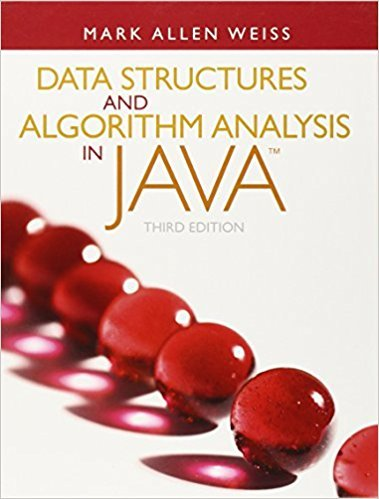×
Get Full Access to Data Structures And Algorithm Analysis In Java - 3 Edition - Chapter 7 - Problem 7.28
Get Full Access to Data Structures And Algorithm Analysis In Java - 3 Edition - Chapter 7 - Problem 7.28

×

# When implementing quicksort, if the array contains lots of duplicates, it maybe betterISBN: 9780132576277 316

## Solution for problem 7.28 Chapter 7

Data Structures and Algorithm Analysis in Java | 3rd Edition

• Textbook Solutions
• 2901 Step-by-step solutions solved by professors and subject experts
• Get 24/7 help from StudySoup virtual teaching assistantsData Structures and Algorithm Analysis in Java | 3rd Edition

4 5 1 250 Reviews
27
1
Problem 7.28

When implementing quicksort, if the array contains lots of duplicates, it maybe better to perform a three-way partition (into elements less than, equal to,and greater than the pivot), to make smaller recursive calls. Assume three-waycomparisons, as provided by the compareTo method.a. Give an algorithm that performs a three-way in-place partition of an N-elementsubarray using only N 1 three-way comparisons. If there are d items equalto the pivot, you may use d additional Comparable swaps, above and beyond the two-way partitioning algorithm. (Hint: As i and j move toward each other,maintain five groups of elements as shown below):EQUAL SMALL UNKNOWN LARGE EQUALi jb. Prove that using the algorithm above, sorting an N-element array that containsonly d different values, takes O(dN) time.

Step-by-Step Solution:
Step 1 of 3

Biol 4305 K= rate of molecular evolution due to drift (for neutral alleles)  = 2Nu * 1/2N  2nu is mutation rate  K=u Example  Pseudogene mutation rate= 1 x 10^-9 mut/yr/bp  How along ago did the ancestor btwn humans and lemurs lived  1 x 10^-9 u/bp/yr x 1000 o 1 x 10^-6 u in 1000bp/yr o ~~ In 10^6 years We have 1 u in 1000bp  150 bp differences o 150 mutations per 10 ^6 years/1 mutation/1000bp/yr or 150 million years of total divergence o 2 branches from one point(putitive ancestors)--> 150 million years of total divergence o Estimate that ~~ the ancestors lived at 75 million years ago (divide it by two from above) In Slides- Test the constancy of the molecular clock  Need phylogeny and DNA sequence data for species and an out group  Estimates of genetic distance Bar graph  4 fold degenerate site- no matter what change happens- it will always change the structure of the amino acids Rate of molecular change graph  Flatter the line- slower the rate of change  Codon position vs rate o First base- asymptote  There is a point that is no longer indicative of the change  Things that have been around for a LONG time - less rater of change  Neutral theory- why we have those patterns of evolution Detecting Selection at molecular level  Neutral theory of molecular evol  Explicative theory for molecular evol  Null hypothesis for empirical theory Neut

Step 2 of 3

Step 3 of 3

##### ISBN: 9780132576277

The full step-by-step solution to problem: 7.28 from chapter: 7 was answered by , our top Science solution expert on 03/02/18, 06:05PM. Since the solution to 7.28 from 7 chapter was answered, more than 242 students have viewed the full step-by-step answer. Data Structures and Algorithm Analysis in Java was written by and is associated to the ISBN: 9780132576277. This full solution covers the following key subjects: . This expansive textbook survival guide covers 12 chapters, and 457 solutions. This textbook survival guide was created for the textbook: Data Structures and Algorithm Analysis in Java, edition: 3. The answer to “When implementing quicksort, if the array contains lots of duplicates, it maybe better to perform a three-way partition (into elements less than, equal to,and greater than the pivot), to make smaller recursive calls. Assume three-waycomparisons, as provided by the compareTo method.a. Give an algorithm that performs a three-way in-place partition of an N-elementsubarray using only N 1 three-way comparisons. If there are d items equalto the pivot, you may use d additional Comparable swaps, above and beyond the two-way partitioning algorithm. (Hint: As i and j move toward each other,maintain five groups of elements as shown below):EQUAL SMALL UNKNOWN LARGE EQUALi jb. Prove that using the algorithm above, sorting an N-element array that containsonly d different values, takes O(dN) time.” is broken down into a number of easy to follow steps, and 120 words.

Unlock Textbook Solution